Courses

# Chapter 9 Inverters - Notes, Power Electronics, Electrical Engineering Electrical Engineering (EE) Notes | EduRev

## Electrical Engineering (EE) : Chapter 9 Inverters - Notes, Power Electronics, Electrical Engineering Electrical Engineering (EE) Notes | EduRev

The document Chapter 9 Inverters - Notes, Power Electronics, Electrical Engineering Electrical Engineering (EE) Notes | EduRev is a part of the Electrical Engineering (EE) Course Electrical Engineering SSC JE (Technical).
All you need of Electrical Engineering (EE) at this link: Electrical Engineering (EE)

INVERTERS

An inverter is a dc-ac converter, i.e. it converters dc supply into ac supply. Evidently the ac output voltage is of desired magnitude and frequency. The output voltage and frequency can be varied as per the requirement of the load.

The DC input supply may come from a battery, solar cells, fuel cells, etc.

Inverters are used in a variety of applications. In small sizes (less than 1000 W or so) they are used in domestic installations as source of standby electric supply. In medium sizes they are used in commercial installation as a source of standby electric supply and uninterruptible power supply (UPS). In medium and large sizes they are used in industrial installations for variable speed AC drives induction heating, etc. One of the biggest application of inverters in HVDC power transmission. At the AC end of this power line AC is converted into DC by controlled rectified. The DC is transmitted over the HVDC line. At the receiving end DC is converted into AC by thyristorised inverters and AC is supplied to consumers.

Classification

Inverters can be classified in a number of ways.

An inverter may be a single phase or 3 phase inverter depending on whether the output is- single phase or 3 phase AC.

Another classification is as per the method of commutation, i.e. line commutated inverter and force commutated inverter. A line commuted inverter feeds an AC system. The AC line voltage is used for commutation. As the AC voltage goes to zero and reverse the thyristor is turned, off. The forced commutated inverters use one of the methods of forced commutation.

Another classification is as per the connections of thyristors and commutating elements. In this category the series inverter, parallels inverter and bridger inverter.

Another classification is voltage source and current inverters. A voltage source inverter is fed by a constant voltage source system with a current source inverter is fed by a constant current source.

In other words, a voltage source inverter has stiff DC voltage source at its input terminals. A currentfed inverter (CFI) or current-source inverter (CSI) is fed with adjustable current from a DC source of high impedance, i.e. from a stiff DC current source. In a CSI fed with stiff current source, output current waves are not affected by the load.

In VSIs using thyristors. some type of forced commutation is usually required. In case FSIs are made up for using GTOs, power transistors, power MOSFETS or IGBTs, self-commutation with base or gate drive signals is employed for their controlled turnon and turn-off.

Single-Phase voltage source inverters: Operating principle :

Single phase bridge inverters are of two types, namely
(1) single-phase half-bridge inverters and
(2) single-phase full-bridge inverters. Basic principles of operation of these two types are presented here.

Power circuit diagrams of the two configurations of single-phase bridge inverters, as stated above, are shown in figure
(a) below for half-bridge inverter and figure (c) for full-bridge inverter. In these diagrams, the circuitry for turning-on or turning-off the thyristors is not shown for simplicity. The gating signals for the thyristors and the resulting output voltage waveforms are shown in figure (b) and (d) for half-bridge and fullbridge inverters respectively. These voltage waveforms are drawn on the assumption that each thyristor conducts for the duration its gate pulse is present and is commutated as soon as this pulse is removed. In figure (b) and (d), ig1 –ig4 are gate signals applied respectively to thyristors T1-T4.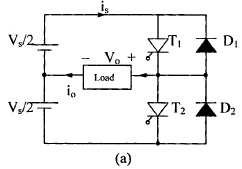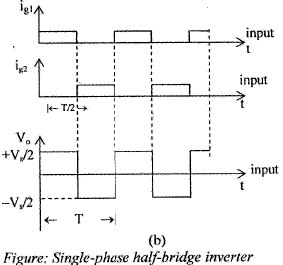Single -phase half bridge inverter, as shown in figure (a consists of two SCRs, two diodes and threewire supply. It is seen from figure (b) that for 0 < t ≤ T/2, thyristor T1 conducts and the load is subjected to a voltage Vs/2 due to the upper voltage source Vs/ 2.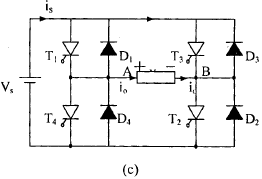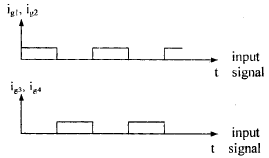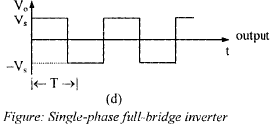At t = 7/2, thyristor T1 is commutated and T2 is gated on. During the period T/2 < t < T, thyristor T2 conducts and the load is subjected to a voltage (– Vs/ 2) due to the lower voltage source (Vs /2). It is seen from figure (b) that load voltage is an alternating voltage waveform of amplitude (Vs /2) and of frequency 1/T Hz. Frequency of the inverter output voltage can be changed by controlling T.

The main drawback of half-bridge inverter is that it require 3-wire DC supply. This difficulty can, however, be overcome by the use of a full-bridge inverter shown in figure (c). It consists of four SCRs and four diodes. In this inverter, number of thyristors and diodes is twice of that in a half bridge inverter.

This, however, does not go against full inverter because the amplitude of output voltage as well as its output power is doubled in this inverter as compared to their values in the half-bridge inverter. This is evident from figure (b) and (d).

In full-bridge inverter, when T1, 72 conduct, load voltage is Vs , and when 73 , 74 conduct load voltage is -Vs as shown in figure (d). Frequency of output voltage can be controlled by varying the periodic time T.

In figure (a), thyristors T1, T2 are in series across the source; in figure (c) thyristors T1, T4 or T3 , T2 are also in series across the source. During inverter operation, it should be ensured that two SCRs in the same branch, such as T1, T4 on figure (c), do not conduct simultaneously as this would lead to a direct short, circuit of the source.

For a resistive load, two SCRs in figure (a) and four SCRs in figure (c) would suffice, because load current io and load voltage Vo would always be in phase with each other. This, however, is not the case when the load is other than resistive. For such types of loads, current io will not be in phase with voltage Vo and diodes connected in antiparallel thyristors will allow the current to flow when the main thyristors are turned, off. These diodes are feedback diodes.

PWM Inverters

Single Pulse Modulation Output voltage of l-f inverter the width pulses in each half cycle is n. If by proper swi of thyristors this pulse width is modulated located symmetrically.

Fourier analysi modulated wave shows that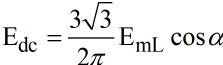Fundamental component V01 = [4Vs/π] sind where nth narmonic

Multiple-pluse Modulation In MPM there several equidistant pulse per half cycle.

Where two pulses per last cycle located symmetrically Fourier analysis of 2- pulse modula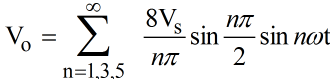nth harmonic content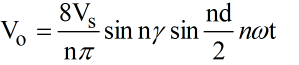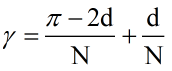where 2d → total PW N → number of pulse per half cycle R.m.s. value of output voltage

Sinusoidal -phase Modulation (sin M): In sin M  technique there are several pulses per half cycle like MPM but each pulse width us bit equal as that in MPM, in sin M the pulse width is a sinusoidal function of the angular position of the pulse.
For realizing sin M, a high frequency triangular carrier wave Vc is compared with a sinusoidal reference wave Vr of designed frequency if triangular wave ø  zero coincide with sinusoid reference zero.

Number of pulse/ half cycle N = fc/ 2f –1 Modulation index

M1 = [Vc/Vc]

MI controls the magnitude of the output voltage.

Disadvantage of FM over PWM scheme

1. Chopping frequency has to be varied over wide range hence filter design becomes difficult.
2. Due to wide range of frequency variation, there is a possibility of interference with signaling and telephone lines in FM scheme.
3. The large OFF time in FM scheme, may make the load current to be discontinuous.

There is one limitation of PWM over FM scheme, low range of a is not possible with PWM as it requires TON to be very low, which is difficult to achieve for commutation circuits.

Offer running on EduRev: Apply code STAYHOME200 to get INR 200 off on our premium plan EduRev Infinity!

65 docs|37 tests

,

,

,

,

,

,

,

,

,

,

,

,

,

,

,

,

,

,

,

,

,

,

,

,

,

,

,

;